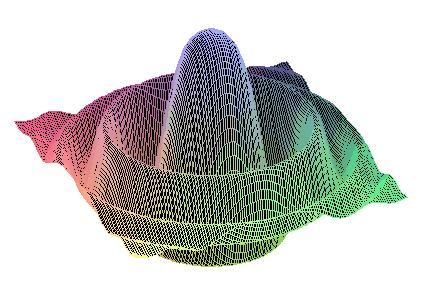DIANA Research Group
DIfferential Algebras and Nonlinear Analysis

Symmetry Groups of Differential Equations

 GF2009    Home    People    Research      Research Topics      Research Projects      START PROJECT      Related Research    Teaching      Current Teaching      Diploma Theses
 Sophus Lie introduced his theory of continuous groups in order to study symmetry properties of differential equations. His approach allowed a unification of existing methods for solving ordinary differential equations as well as classifications of symmetry groups of partial differential equations. Here a symmetry group of a differential equation is a Lie-group action on some space of independent and dependent variables transforming solutions of a given PDE into other solutions. Lie's methods have been developed into powerful tools for examining partial differential equations through group analysis. In many cases, symmetry groups are the only known means for finding concrete solutions to complicated equations. Moreover, group analysis of differential equations has revealed far- reaching connections between symmetry groups of PDEs and conservation laws of mathematical physics (Nöther-theorems). Recently, the immense amount of computations needed for determining symmetry groups of concrete systems has been greatly reduced by the implementation of computer algebra packages for symmetry analysis of PDEs. Our interest in group analysis of differential equations lies in a synthesis of symmetry methods with results from the theory of algebras of generalized functions. A description of our research in this direction can be found at M. Kunzinger's research page.
Last changed January 3, 2007 by M.K. and R.S.